﻿ 高阶线性常微分方程零点稳定性的直接判定法 Direct Method of Stability of Null Solution for Higher Order Ordinary Differential Equations

Vol.05 No.03(2016), Article ID:18406,4 pages
10.12677/AAM.2016.53051

Direct Method of Stability of Null Solution for Higher Order Ordinary Differential Equations

Zhixiang Wang, Yuedan Jin, Xiangqing Zhao

Department of Mathematics, Zhejiang Ocean University, Zhoushan Zhejiang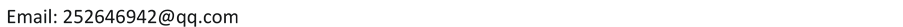Received: Jul. 27th, 2016; accepted: Aug. 18th, 2016; published: Aug. 25th, 2016ABSTRACT

“Higher order ordinary differential equations can always be transformed to be first order ordinary differential systems”. By this fact, we proved that higher order ordinary differential equation and first order ordinary differential system transformed from it have the same characteristic roots. Then, by Lyapunov Theorem, we show that the stability of null solution of higher order ordinary differential equation can be determined by its characteristic roots.

Keywords:Higher Order Ordinary Differential Equation, First Order Ordinary Differential System, Stability of Null Solution, Characteristic Roots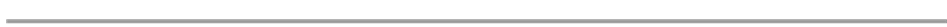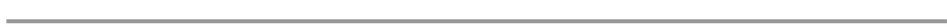“高阶线性常微分方程都可以化成一阶线性常微分方程组”。证明了高阶线性常微分方程与由它转化所得的一类特殊的一阶线性常微分方程组有相同的特征值，并据此利用李雅普诺夫定理证明了“高阶线性常微分方程零解的稳定性可以由它的特征值直接决定”。1. 引言(1.1)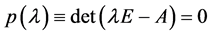(1.2)

2. 高阶方程与一阶线性方程组(2.1)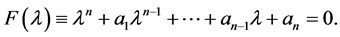(2.2)3. 高阶线性常微分方程零解稳定性直接判定法1)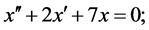2)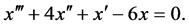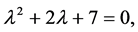(3.3)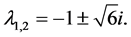2) 三阶线性常微分方程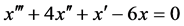的特征方程为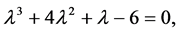(3.4)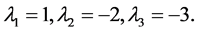Direct Method of Stability of Null Solution for Higher Order Ordinary Differential Equations[J]. 应用数学进展, 2016, 05(03): 412-415. http://dx.doi.org/10.12677/AAM.2016.53051

1. 1. 王高雄, 朱之铭, 周思铭, 等. 常微分方程(第三版) [M]. 北京: 高等教育出版社, 2010.

2. 2. 丁同仁, 李承治. 微分方程教程[M]. 北京: 高等教育出版社, 2002.

3. 3. Perko, L. (2001) Differential Questions and Dynamical Systems. 3rd Edition, Sprin-ger-Verlag, Berlin Heidelberg. http://dx.doi.org/10.1007/978-1-4613-0003-8